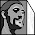## Wednesday, June 1, 2011

### Functions and Iterations, and how fractals are generated

To start, let me show you a few images of Julia set fractals.Beautiful, aren't they? But how on earth do we get to these amazing images, from the very simple-seeming mathematics previously described? Well, let's continue...

Ok, so in the previous post you learnt about what complex numbers are, and how to multiply them. But knowing this isn't really the most interesting thing in the world. What is interesting is what happens when you take any complex number and feed it into some function.

First, what is a function? Simply, it is a mathematical formula that takes your input, and changes it in some way, to give an output. And an iteration? It's simply executing that same function again, but with the resulting output from the previous calculation. Take a look at this, using the function I've previously mentioned that is responsible for almost all fractal shapes ( f(z)= z² + c ):

Let's take any point on the complex plane, i.e. any complex number, let's say, (-1.5, 2i)
If you feed that into our function, with c=0 for now, you get:

f(-1.5, 2i) = (-1.5, 2i
= (-1.5, 2i)(-1.5, 2i)
= 2.25 - 3i -3i - 4
=(-1.75 - 6i)

So the output is (-1.75 - 6i). Using this output, the next iteration is:

f(-1.75 - 6i) = (-1.75 - 6i)² to which the answer, or output is (-32.9375 + 21i)
And the next iteration:
f(-32.9375 + 21i) = (-32.9375 + 21i

etc

Now, how does this look on a graph? Here:
Since the answer to the next iteration is (643.87890625 - 1383.375i), I'm sure you understand why I didn't go further on the graph. But now, what does all this mean in regards to fractals? ... We're getting there.

What you need to understand is that, when you feed any complex number into the function f(z)= z² + c, and reiterate the function forever, and map each resulting output point, a number of things can happen. It might map out  to become forever and ever larger, until eternity, which is called escaping to infinity. It might become stuck in a cycle. It might eventually simply stop. It might also spiral down to zero. Here's a diagram to help illustrate a few of the different possible "fates" of a point when forever reiterated in a function and mapping each resulting output point from one to the next:
But essentially, a point does either one of two things: It either escapes to infinity, or, it stays bound.

And this, dear readers, is the key to how Fractals are made. This is what is done:

A fractal generator program takes every possible complex number on the complex plane, and colours it, depending on whether it escapes to infinity, or stays bound, after being eternally reiterated in a function. The points that stay bound are coloured black. The points that escape to infinity are coloured according to "how fast" they escape to infinity. The colours are entirely customisable.

Let's take our function,  f(z)= z² + c, make c = 0, enter it into a fractal generator (Fractal eXtreme) and see what happens:

Not very exciting is it? But believe it or not, you're looking at the simplest form of the Julia set fractals. The center of the circle is point zero on our number line, and its radius = 1.

Now what will happen if we change the value of c? ... For example, let's makes c 0.31 and see what happens when we feed the same function into Fractal eXtreme:

Much better, wouldn't you agree? :) So much more interesting. And now, instead of making c simply a real number, let's make it a complex number... For example (0.31, 0.5i) ... And what do we get?:

So this is the fun part :) Download Fractal eXtreme and experiment a bit with different numbers. To enter different numbers, when the program opens, you need to choose New, and then check the little box next to "Julia" ... When the fractal loads, a box with X and Y as input fields will appear. X is the real number and Y is the imaginary number which form the complex number 'c' in our function. Notice that if you make them both = 0 then the Julia generated is the circle I showed you above.

Lastly, here are the two videos I originally learnt this all from. So if you're feeling kinda lost right now (it can be a lot to take in), first, reread my post, and then, watch and rewatch these two videos, until you understand. Thanks for your attention and goodnight!

Complex Plane Dynamics:

How Julia Set Fractals are Generated:

1.Nice, about a year ago I became extremely passionate about fractals and fractal math. Since then, I've dug deeper and deeper whenever I had the opportunity. Thanks so much for this, and a great blog. I look forward to reading more.

1.Hi Vinnie =)

Thank YOU, for taking the time to comment. I, too, suddenly got into fractals at one point and dug into them with zest, hehe. You might see my one blogpost showing off my mandelbrot tattoo ^^ ... Please keep me updated with your progress in the infinite fractal world =)

2.Absolutely informative, thank you very much. I just recently got into fractal math about a year and a half ago. I've mostly just been playing with it and just opened into the 3D mandelbulb sets a few months ago. I've reached what I feel is a plateau as far as random exploration with things I don't quite understand so I've decided to actually learn the math behind the fractals and how they're generated so I can actually create instead of just exploring haphazardly - though ironically enough that's kind of how they're generated anyway, as I understand, through systematic chaotic iterations. Nifty nifty neat stuff.